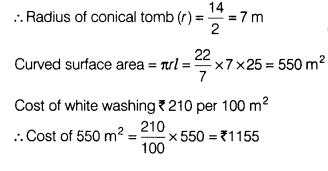# The slant height and base diameter of a conical tomb are 25 m and 14 m respectively

The slant height and base diameter of a conical tomb are 25 m and 14 m respectively. Find the cost of white washing its curved surface area at the rate of ^ 210 per 100 m2?

Given, diameter of conical tomb = 14m
and slant height of conical tomb (/) = 25 m